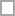# Multiplying Polynomial Quizzes & Trivia

Questions: 4  |  Attempts: 272   |  Last updated: Jan 22, 2013
• Sample Question
Simplify:  -6(x + 9)A polynomial is an equation with many terms. Some people face problems when it comes to working out math problems when it comes to polynomials especially multiplication. Are you one of them? Give the quiz below a try and let...

Questions: 9  |  Attempts: 226   |  Last updated: Jun 25, 2018
• Sample Question
2.  Multiply.Questions: 5  |  Attempts: 54   |  Last updated: Dec 9, 2019

3.1b Algebraic Connections - Multiplying Polynomials (ICHIHANA)

Questions: 15  |  Attempts: 9   |  Last updated: Feb 12, 2013Related

## Multiplying Polynomial Questions and Answers

• Simplify:  -6(x + 9)Multiplying polynomial question from

• Simplify this expression as much as you can:  (x + 7)(x + 3)Multiplying polynomial question from

• Check ALL of the expressions that are equal to: (x + 2)(x - 8)Multiplying polynomial question from

• 2.  Multiply.Multiplying polynomial question from

• 4.  Multiply.Multiplying polynomial question from

• 8.  Multiply.Multiplying polynomial question from

•Multiplying polynomial question from

•Multiplying polynomial question from

•Multiplying polynomial question from